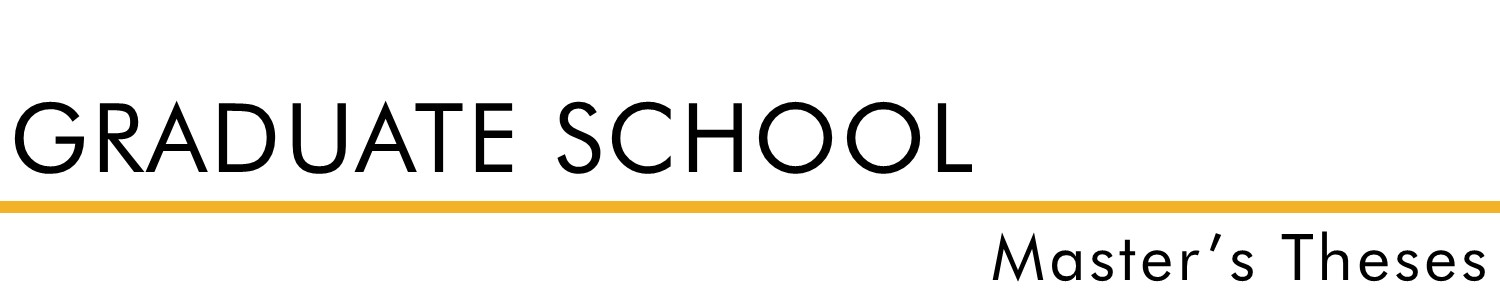#### Document Type

Thesis - campus only access

Summer 1953

#### Degree Name

Master of Science (MS)

#### Department

Math

Dr. Emmet Stopher

#### Abstract

Mathematics is a science complete in itself . In studying mathematics the use of some kind of mathematical tables will be needed for certain types of courses . The tables available will not always be sufficient to give the desired results . This will necessitate some kind of interpolation to give the information wanted. Interpolation is the art or science of "reading between the lines of mathematical tables . 11 According to the definition, interpolation is the insertion of mathematical terms according to the law of the function involved. 1 The kind of interpolation usually used, since it is the common one, is linear or straight line interpolation . This method uses the value directly above and below the desired result, finding the difference between these tabulated values, taking the proportional part thereof, and adding this t o the smaller given value . This result is usually sufficiently accurate for work on the high school level since accuracy to four decimal points is wanted . The approximate answer thus obtained is due partly to the fact that when these two values are so used they do not necessarily trace the function involved but divert it into a straight line . When the study of this problem was undertaken it was the normal assumption that parabolic and cubic interpolation would give a more accurate result than the common conventional straight line interpolation . The purpose of this thesis is to investigate the extent of the superiority of parabolic interpolation over straight 2 line interpolation and cubic interpolation over parabolic interpolation. It is t he objective to do this by means of theorems, equations of existing formulas, and by compiled tables . This study is limited in scope to three methods of interpolation as applied to the sine function between zero and ninety degrees. The methods of interpolation used are three: straight line interpolation, parabolic interpolation, and cubic interpolation. The table developed for this investigation is taken at four different points of the range . Values are obtained near zero, thirty, sixty, and ninety degrees . The widths of the intervals used are constant, meaning that the control points are evenly spaced. Equal intervals are used because mathematical tables give trigonometric functions at uniform intervals .

For questions contact ScholarsRepository@fhsu.edu